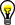# DDC Enabled

NI High-Speed Digitizers Help (NI-SCOPE)

Edition Date: January 2017

Part Number: 370592AB-01

View Product InfoNI-SCOPE (English | Japanese)

The DDC Enabled property/attribute activates the functionality of the DDC block. To use any of the features in the DDC block, you must set DDC Enabled to TRUE.

LabVIEWC/C++
DDC EnabledNISCOPE_ATTR_DDC_ENABLEDTip  To achieve maximum flatness in the filter response of the device in DDC mode, set the maximum input frequency to full bandwidth (–1). However, keep in mind that this action does not protect against aliasing frequency content of the input signal above (0.5 × Sample Clock Timebase Rate). When using internal clocking, the Sample Clock Timebase Rate is 100 MS/s (NI 5142) or 150 MS/s (NI 5622).

## Hardware Calibration of Binary Data

In a normal acquisition, you cannot assume that the binary data maps perfectly to the vertical range. For example, if you fetch 16-bit binary data, you might expect that the positive full scale binary value corresponds to the maximum positive voltage of the vertical range, and that the negative full scale binary value corresponds to the maximum negative voltage. This is not the case, however, for a number of reasons. First, the full scale of the ADC is not mapped to the vertical range. A few codes on the positive and negative ends are left as headroom, in case the input signal slightly exceeds the specified vertical range. Second, the binary values do not account for the required corrections to gain and offset based on calibration data. If you fetch binary data, you can convert to voltage values using the gain and offset values from the wfmInfo struct. These values account for the ADC normalization and calibration data.

Voltage = (Binary Value × wfmInfo.gain) + wfmInfo.offset

When the DDC is enabled, the onboard signal processing block accounts for both ADC normalization and calibration data. So the waveform samples are stored and can be fetched as calibrated, normalized binary data. This means that the range of binary values maps exactly to the vertical range you configure.

Voltage = Binary Value × Range/2(ADC resolution in bits)

The gain and offset values in the wfmInfo struct work just as well in this case (but they do not hold normalization and calibration information), so the following conversion still works:

Voltage = (Binary Value × wfmInfo.gain) + wfmInfo.offset

In a normal acquisition, you must know the gain and offset values to correctly convert the binary data to voltage values. In DDC mode, you can assume the following:

• The maximum positive binary value maps to the maximum positive voltage of the vertical range.
• The maximum negative binary value maps to the maximum negative voltage of the vertical range.
• The vertical range is divided evenly across the possible binary values.

These assumptions may be useful for streaming or logging binary data, because you do not need to know the gain and offset value to recover the measured voltages.